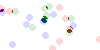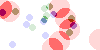Is it possible to apply dynspread (or spread) based on a categorical variable? E.g., cat A gets spread or dynspread by 2 pixels, whereas cat B does not?

Thanks!

Yes you can. A categorical aggregation such as returned by `Canvas.points` is 3D of shape `(ny, nx, ncategories)`. If you pass this to `tf.spread` then it performs the spread separately on each category, and you would combine and colorize this using `tf.shade`. Hence it is possible to extract just one category from the aggregation, pass it to `tf.spread` on its own with a different pixel spread, then combine it with the original `spread` result. This is best illustrated with an example:

``````import datashader.transfer_functions as tf
import numpy as np
import pandas as pd

n = 24
rng = np.random.default_rng(1231)
df = pd.DataFrame(data=dict(
x=rng.uniform(size=n),
y=rng.uniform(size=n),
cat=np.repeat(['a', 'b', 'c'], n // 3),
))
df["cat"] = df["cat"].astype("category")
color_key = dict(a="red", b="green", c="blue")

cvs = ds.Canvas(plot_height=50, plot_width=100,x_range=(0, 1), y_range=(0, 1))
agg = cvs.points(source=df, x="x", y="y", agg=ds.by("cat"))
``````

This is standard use if `spread` and `shade` to giveNow to `spread` category ‘a’ more than the others:

``````a_only = tf.spread(agg.loc[dict(cat='a')], px=10)
agg2.loc[dict(cat='a')] = a_only